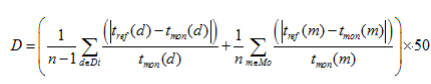## Calculate the false rejection, Computer Network Security

Assignment Help:

Divide the user data into 6 equal sets. Use the first set for the enrollment phase of your system, and the rest for the verification phase. Use the following formula to calculate the deviation (D) between the enrollment phase time data (also referred to as a  target or reference time) and the verification phase time data, (also known as a monitored time) for the two columns representing the Fly Times and the Dwell Time. You can use either of the First Key Dwell Time column values or the Second Key Dwell Time column values in your data set, however you have to use the same column throughout your implementation.Where | | denotes the absolute value function, tref is the targeted or reference time for dwell or fly time values (from the enrollment phase data) and tmon is the monitored time for dwell or fly time values (from the verification phase data), Di is the set of fly time values in the sample, Mo is the set of dwell time in the sample, and n is the size of the sample. The type of n (as well as the sizes of Di and Mo) should be declared as a variable in your implementation, but it should be assigned the maximum possible value of 500.

• To calculate the False Rejection (FR) for a given user, compare each of the five verification session data of the same user against the enrollment set data by calculating the deviation for the fly times and dwell time. Note that this will produce '5' different values of D (one for each set of verification session data). For each of those of D's, if D>=Thr, FR=1 else FR=0, where D is the calculated value for the deviation and Thr is the value of threshold%.
• To calculate the False Acceptance (FA) for the same user (as above), compare each of the verification session data of the 4 remaining users against the enrollment set data of the same user by calculating the deviation for the fly times and dwell time. Note that this will produce '5*4=20' different values of D (one for each set of verification session data for each different user). For each of those D's, if D<= Thr, FA=1 else FA=0, where D is the calculated value for the deviation and Thr is the value of threshold%.

Now that you have got the values of FA and FR for this user, you can calculate the  False Acceptance Rate (FAR) and the False Rejection Rate (FRR) for this user by computing:

FAR=Sum of FA/total number of verification trials
FRR=Sum of FR/Total number of verification trials

If both computed FAR and  FRR  values are <= 0.4, then the FAR and the FRR conditions are satisfied. In this case, the algorithm should printout 'Access Granted! and list the file (name and location) accessible and what kind of access right to those file the user has. If the FAR and the FRR conditions fail, the message could be  'Access Failed! Your attempt and corresponding IP used will be reported to the system administrator."

#### Quote, How much would it cost to have a project completed by tomorrow night...

How much would it cost to have a project completed by tomorrow night?

#### Find the capacity of the wcdma, Question: (a) Describe the term interfe...

Question: (a) Describe the term interference in the space, time, frequency, and code domain. (b) Consider a 1 G - AMPS: 824-849 MHz (forward) ; 869-894 MHz (reverse). B

#### Hybrid cryptography systems-cryptography, Hybrid Cryptography Systems Th...

Hybrid Cryptography Systems This makes use of different cryptography systems. Except digital certificates, pure asymmetric key encryption is not used extensively. Asymmetric enc

#### Access control matrix, You are provided with 3000 sample keystrokes for 5 u...

You are provided with 3000 sample keystrokes for 5 users mentioned above. You can find this data using the ‘Lab 2 User Data' link in the ‘Lab 2' folder on Blackboard. Please note t

#### What is feedforward multilayer perceptron neural network, Question: (a)...

Question: (a) Explain and illustrate with a drawing the three-layer feedforward multilayer perceptron neural network. (b) Summarize the standard backpropagation algorithm in

#### Computer science, What is the use of it

What is the use of it

#### Direct point-to-point communication:, Early networks used simple point-to...

Early networks used simple point-to-point communication . In such a method of communication every communication channel connects exactly two devices. In this way it prepares a m

#### Arp message format, ARP MESSAGE FORMAT Although the ARP data packet fo...

ARP MESSAGE FORMAT Although the ARP data packet format is sufficiently general to allow hardware addresses and arbitrary protocol. ARP is almost usually used to bind a 32-bit

#### Explain any two types of security policies, Question 1 Explain any two typ...

Question 1 Explain any two types of security policies Question 2 What is security attack? Explain with examples Question 3 Explain different characteristics that i

#### Network, what is computer network?

what is computer network?